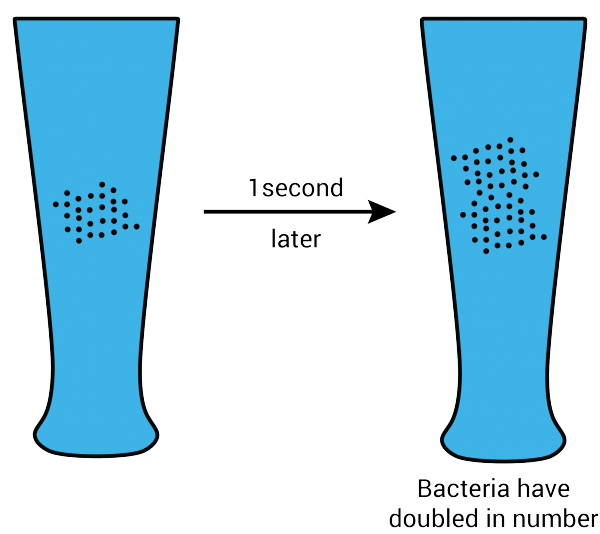Math Puzzles

# PUZZLE SOLUTION P4

1.3k views

Half a minute is not the answer. If we reason and think, we can easily arrive at the answer.

Let’s have a look at the question:

A number of bacteria are placed in a glass. One second later, each bacterium divides in two, the next second each of the resulting bacteria divides in two again, and so on. After one minute the glass is full. When was the glass half empty?After every second, the number of bacteria in the glass doubles. Since by the end of 1 minute the glass is full, the previous second, it would be half the number.

Therefore, after the 59th second, the glass would be half empty.

Congratulations if you got the correct answer!

Related Articles
Award-winning math curriculum, FREE for a year